# Can Velocity Be Negative? (What It Means & 3 Key Ideas)

Velocity is used in math and physics to describe how the position of an object changes.  However, it can be a little tricky to navigate the signs (positive and negative) of velocity as it relates to position and acceleration.

So, can velocity be negative?  Velocity can be negative when position is decreasing. This happens when an object moves in a negative direction. Negative velocity & negative acceleration means increasing speed (in a negative direction). Negative velocity & positive acceleration means decreasing speed (also called deceleration).

Of course, negative velocity is not theoretical, since it occurs in falling objects and other natural phenomena.

In this article, we’ll talk about negative velocity and when it can happen.  We’ll also look at some examples to make the concept clear.

Let’s get started.

Hey! If you need to learn more about velocity and other math concepts for physics, check out this course:

Advanced Math For Physics: A Complete Self-Study Course

## Can Velocity Be Negative?

Velocity can be negative in physics or calculus, such as when an object is in free fall (due to the effects of gravity).  Speed is the absolute value of velocity, which gives us a nonnegative number with no direction.

Velocity is negative whenever position is decreasing.  This is because velocity is the derivative of position.

That is, velocity tells us how position is changing (how quickly an object is moving, and in which direction).  Negative velocity means position is decreasing, and positive velocity means position is increasing.

It is important to note that negative acceleration does not always mean a decrease in velocity or speed.  Since speed is the absolute value of velocity, we could have increasing or decreasing speed when acceleration is negative.

The table below helps to illustrate what happens with acceleration, velocity, and speed when they have different signs.

As the table above suggests, negative velocity and negative acceleration means an object is speeding up: as the velocity becomes more negative, its absolute value (speed) increases.  We are really just speeding up in the negative direction.

Do not confuse deceleration with negative acceleration! Deceleration means a reduction in speed.

### What Does Negative Velocity Mean?

Negative velocity means decreasing position.  Negative velocity indicates one of the following:

• The object is moving in a negative direction, and it is slowing down.  [negative velocity, positive acceleration, decreasing speed]. An example would be a rocket falling (negative velocity) firing retro thrusters (positive acceleration) to slow its descent (decreasing speed).   This is an example of deceleration.
• The object is moving in a negative direction, and it is speeding up.  [negative velocity, negative acceleration, increasing speed]. An example would be a bird flying towards the water (negative velocity) flapping its wings to fly downwards faster (negative acceleration) to speed its descent (increasing speed) to catch fish.

As you can see, negative velocity does not always indicate a decrease in speed.  We would also need to know the sign of the acceleration.

### What Does Negative Velocity Look Like On A Graph?

Negative velocity looks like a decreasing function on a graph of position.  This is because velocity is the first derivative of position, and a negative first derivative indicates a decreasing function (as we move left to right on the graph, the function moves downward).

The graph of the position of an object with negative velocity and zero acceleration would be a line with a negative slope, such as the one below, but there are other possibilities.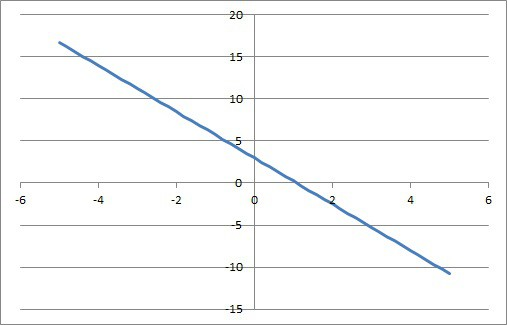An object with negative velocity (and constant acceleration) will have a position graph that looks like a line with a negative slope (a decreasing function of time).

The graph of the velocity of an object with negative velocity but zero acceleration would look like a horizontal line y = k for a negative value of k (such as the one shown below).  This is because acceleration is the first derivative of velocity, and a zero first derivative indicates a constant function.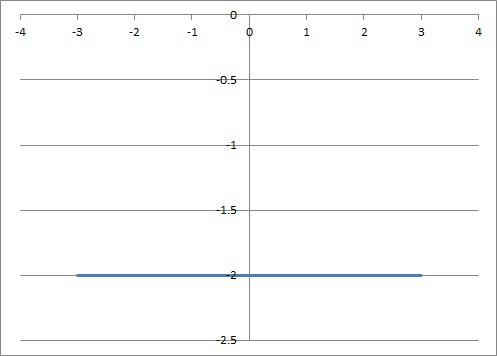This horizontal line would be the graph of the velocity of an object with negative velocity and zero acceleration.

### What Is The Difference Between Positive & Negative Velocity?

The difference between positive and negative velocity is the sign or direction of the change in position:

• Positive velocity means that position is increasing (moving in the positive direction, or up).
• Negative velocity means that position is decreasing (moving in the negative direction, or down).

### Can Velocity Be Negative & Acceleration Positive?

Velocity can be negative when acceleration is positive.

Negative velocity and positive acceleration means that an object is moving in a negative direction (due to negative velocity), and it is slowing down (due to positive acceleration).

The graph of such a position function would be a decreasing part of a convex curve, such as the one in the image below.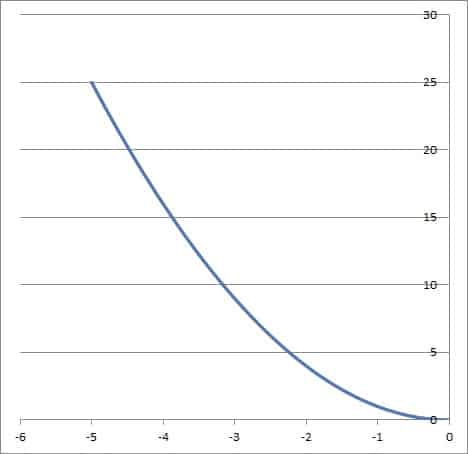This position graph shows positive acceleration (concave up curve) and negative velocity (decreasing curve). The object is moving in a negative direction, but at a slower and slower pace.

#### Example Of Negative Velocity & Positive Acceleration

An example of negative velocity and positive acceleration would be a rocket falling to the ground (negative velocity) and using retro thrusters (positive acceleration) to slow its descent to Earth.A rocket falling towards a planet (negative velocity) but using retro thrusters (positive acceleration) to reduce speed for impact.

#### Example Of Negative Velocity & Negative Acceleration

An example of negative velocity and negative acceleration would be a bird diving towards the water (negative velocity) and beating its wings (negative acceleration) to speed up in the negative direction as it tries to catch fish in the water.A tern will have negative velocity as it moves down towards the water, and its acceleration will be negative if it flaps its wings to speed up as it descends.

### What Is An Example Of Negative Velocity?

One of the most common examples of negative velocity is a falling object.  Since a falling object has a decreasing height (vertical position), its velocity will be negative (unless it has some sort of upward lift, such as a helicopter’s blades spinning).

When an object is dropped from rest, it accelerates (in the negative or downward direction), leading to a negative velocity.  The acceleration due to gravity is -9.8 m/s2 (or -32 ft/s2).

When dropped from rest, an object’s velocity will decrease by 9.8 m/s per second (or 32 feet per second), due to the acceleration of gravity.

So, the object’s velocity would be:

• -9.8 meters per second after falling for 1 second
• 2*(-9.8) = -19.6 meters per second after falling for 2 seconds
• 3*(-9.8) = -29.4 meters per second after falling for 3 seconds
• … and so forth

You can see the graphs of position and velocity of an object dropped from rest in the graphs below.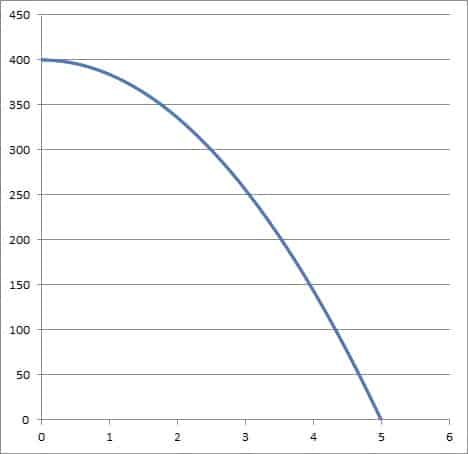This graph shows the position (height) graph of an object dropped from rest at a height of 400 feet and falling for 5 seconds. The acceleration due to gravity is -32 feet per second per second.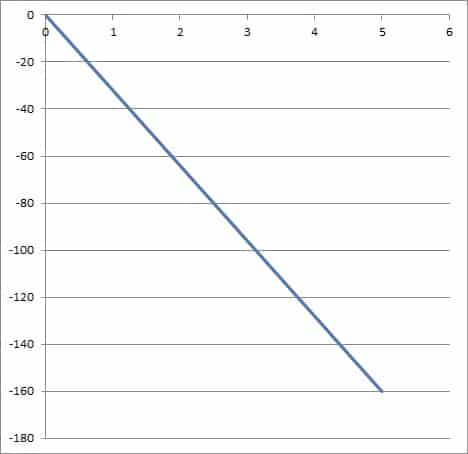This graph shows the velocity of an object dropped from rest at a height of 400 feet and falling for 5 seconds. The acceleration due to gravity is -32 feet per second per second. The velocity of the object when it hits the ground is -160 feet per second.

### Can Average Velocity Be Negative?

Average velocity can be negative over a time interval.  All that is required is that the final position is less than the starting position.

To prove this, remember that the formula for average velocity over a time interval is:

• vavg = (pf – p0) / (tf – t0)

where t0 is the starting time, tf is the final time, p0 is the starting position (at time t0), and pf is the final position (at time tf).

Since the final time will be after the starting time, we know that the denominator tf – t0 will always be positive.

That means that the average velocity will be negative if and only if the numerator is negative, or if:

• pf – p0 < 0
• pf < p0

That is, if the final position pf is less than the starting position p0.

From a practical perspective, if an object’s net movement is down or backwards (in a negative direction) over a time interval, then its average velocity will be negative.

For example, if I climb up 10 feet and then down 40 feet, my net change in position is (+10) + (-40) = -30 feet.  If it took me 10 minutes to make this progress, my average velocity is -30 / 10 = -3 feet per minute.

#### Example: When Average Velocity Is Negative

Let’s say that a car has a starting position of 65 miles north of Boston at t = 1 hour.

At t = 6 hours, the car has a final position of 35 miles north of Boston.

To find the average velocity over this time interval, we apply our formula from before:

• vavg = (pf – p0) / (tf – t0)
• vavg = (35 –65) / (6 – 1)
• vavg = (-30) / (5)
• vavg = -6

So, the average velocity over this time interval is -6 miles per hour.

That means that, on average, the car’s position decreased by 6 miles for every hour we were driving (we got 6 miles closer to Boston per hour, on average).

Since there are 60 minutes in an hour, we could also say that on average, the car’s position decreased by 1 mile per hour every 10 minutes (1 mile closer to Boston every 10 minutes, on average).

Of course, we don’t know how often the car changed speed between the starting and ending points (acceleration).  It could have stopped completely, gone faster or slower than -6 miles per hour, or both.